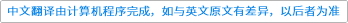International Journal of Mathematics ( IF 0.604 ) Pub Date : 2021-04-10 , DOI: 10.1142/s0129167x21500373
Inyoung Park, Jian Zhao, Kehe Zhu

For positive $p$ and real $α$ let $Aαp$ denote the weighted Bergman spaces of the unit ball $𝔹n$ introduced in [R. Zhao and K. Zhu, Theory of Bergman Spaces on the Unit Ball in$ℂn$, Mémoires de la Société Mathématique de France, Vol. 115 (2008)]. It is well known that, at least in the case $n=1$, all functions in $Aαp$ can be approximated in norm by their Taylor polynomials if and only if $p>1$. In this paper we show that, for $f∈Aαp$ with $0, we always have $∥SNf−f∥p,β→0$ as $N→∞$, where $β>α+n(1−p)$ and $SNf$ is the $N$th Taylor polynomial of $f$. We also show that for every $f$ in the Hardy space $Hp$, $0, we always have $∥SNf−f∥p,β→0$ as $N→∞$, where $β>n(1−p)−1$. This generalizes and improves a result in [J. McNeal and J. Xiong, Norm convergence of partial sums of $H1$ functions, Internat. J. Math.29 (2018) 1850065, 10 pp.].down
wechat
bug